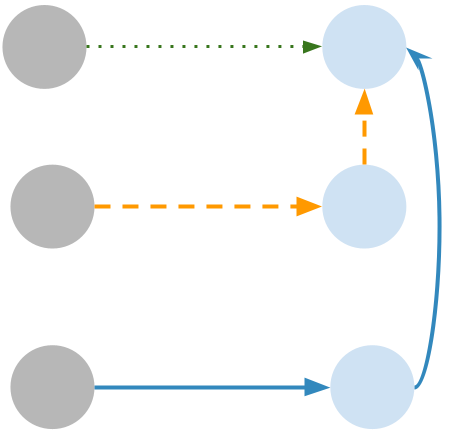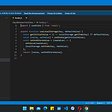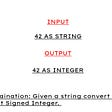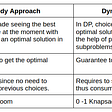# Why this problem is interesting to me

• This is the first problem I can use DP, BFS and Dijkstra to solve
• This is the first problem that I can use Deque (replace the Priority queue) to implement the Dijkstra to achieve O(V) complexity (recall the regular complexity of Dijkstra is O((V+E)log V).

# DP

We can use DP to solve this problem. The state transitions can be found in the following image.State transitions. The first path has 0 extra costs, the rest two paths have an extra cost of 1 for each.

## Code

usually we use i for row and j for column. In this case, we flip it and use i as the first layer loop which is in the column direction.

`int f;const int INF = 0x3f3f3f3f;class Solution {public:    int minSideJumps(vector<int>& b) {        memset(f, 0x3f, sizeof f);        f = 0;        f = f = 1;        const int n = b.size();        for (int i = 1; i < n; ++i) {            for (int j = 0; j < 3; ++j) {                // when obstacle, continue                if (b[i] == j + 1) continue;                for (int k = 0; k < 3; ++k) {                    int cost = 0;                    // if the path contain side jump, set cost to 1.                    if (k != j) cost = 1;                    // if the corresponding path has obstacle, continue                    if (b[i] == k + 1) continue;                    f[i][j] = min(f[i][j], f[i-1][k] + cost);                }            }        }        return *min_element(f[n-1], f[n-1] + 3);    }};`

run time

`Runtime: 288 ms, faster than 80.65% of C++ online submissions for Minimum Sideway Jumps.Memory Usage: 193.9 MB, less than 56.93% of C++ online submissions for Minimum Sideway Jumps.`

# BFS

We can use BFS to find the shortest path if every edge’s cost is equal. In this case, the cost can only be 0 and 1, we can still use BFS to achieve our goal: finding the shortest path.

Recall the regular BFS

1. this_level {node1, node2…}, depth = d
2. next_level = {neighbor of node for node in this_level if neighbor is not visited}, depth++

Of course, you can use queue, the idea is the same.

For this problem, let’s do slightly modification

1. this_level {node1, node2…}, depth = d
2. Add all zero cost reachable nodes to this_level as the cost is zero, it will not introduce extra cost. We look right for each node, and add valid nodes to this_level.
3. next_level = {neighbor of node for node in this_level if neighbor is not visited}, depth++

Code

`const int N = 500010;int visited[N];class Solution {public:    int minSideJumps(vector<int>& b) {        memset(visited, 0, sizeof(visited));        const int n = b.size();        vector<pair<int, int>> this_level;        this_level.emplace_back(0, 1);        visited = 1;        int depth = 0;        while (true) {            int len = this_level.size();            // For each element, see whether can jump to the right as this has 0 cost. If so, put it in the current level.            for (int idx = 0; idx < len; idx++) {                auto [i, j] = this_level[idx];                while (i + 1 < n && !visited[i+1][j] && b[i+1] != j +1) {                    if (i + 1 == n -1) return depth;                    visited[i+1][j] = 1;                    this_level.emplace_back(i+1, j);                    i++;                }            }            vector<pair<int, int>>next_level;            // check whether it can have a side jump. If so, put in next level.            for (auto& [i, j] : this_level) {                for (int k = 0; k < 3; ++k) {                    if (k != j && b[i] != k + 1 && !visited[i][k]){                        visited[i][k] = 1;                        next_level.emplace_back(i, k);                    }                }            }            this_level = next_level;            depth++;        }        return 0;    }};`

run time

`Runtime: 708 ms, faster than 19.90% of C++ online submissions for Minimum Sideway Jumps.Memory Usage: 284 MB, less than 13.61% of C++ online submissions for Minimum Sideway Jumps.`

# Naive Dijkstra by using priority queue

We can naively apply the dijkstra algorithm. Dijkstra algorithm has the complexity of O((V + E)log V). We still can get the code AC.

Code

`typedef pair<int, int> PII;const int N = 500010<<2;int dist[N];class Solution {public:    int minSideJumps(vector<int>& b) {        const int n = b.size();        // set dist as INF        memset(dist, 0x3f, sizeof(dist));        // use (i<<2) + j to encode i and j.        dist = 0;        priority_queue<PII, vector<PII>, greater<PII>> pq;        pq.emplace(0, 1);        while (!pq.empty()){            auto [d, u] = pq.top(); pq.pop();            if (d > dist[u]) continue;            auto i = u>>2, j = u&3;            for (int k = 0; k < 3; k++) {                // go right                if (j == k && i + 1 < n && b[i+1] != k + 1) {                    auto v = ((i+1)<<2) + k;                    if (dist[u] >= dist[v]) continue;                    dist[v] = dist[u];                    pq.emplace(dist[v], v);                }                 if (j != k && b[i] != k + 1) {                    auto v = (i<<2) + k;                    if (dist[u] + 1 >= dist[v]) continue;                    dist[v] = dist[u] + 1;                    pq.emplace(dist[v], v);                }            }       }       return min(dist[(n-1)<<2], min(dist[((n-1)<<2) + 1], dist[((n-1)<<2) + 2]));    }};`

Run time

`Runtime: 664 ms, faster than 22.43% of C++ online submissions forMinimum Sideway Jumps.Memory Usage: 195.9 MB, less than 54.88% of C++ online submissions for Minimum Sideway Jumps.`

# Improved Dijkstra by using Deque.

This is essentially a special case of the Dijkstra algorithm. We only have 2 cost values, 0 or 1. We put 0 at the beginning and 1 at the end, which is essential a priority queue as:

Priority queue is popping the node with smallest cost. The smallest cost + an edge with 0 cost is still the smallest cost node. It can be push_front of the queue.

For this code, as we are using the deque and each node is put into the deque only one time, the complexity is O(V) where V is 3*n.

## Code

`typedef pair<int, int> PII;const int N = 500010<<2;int dist[N];class Solution {public:    int minSideJumps(vector<int>& b) {        const int n = b.size();        // set dist as INF        memset(dist, 0x3f, sizeof(dist));        // use (i<<2) + j to encode i and j.        dist = 0;        deque<PII> dq;        dq.emplace_front(0, 1);        while (!dq.empty()){            auto [d, u] = dq.front(); dq.pop_front();            if (d > dist[u]) continue;            auto i = u>>2, j = u&3;            for (int k = 0; k < 3; k++) {                // go right                if (j == k && i + 1 < n && b[i+1] != k + 1) {                    auto v = ((i+1)<<2) + k;                    if (dist[u] >= dist[v]) continue;                    dist[v] = dist[u];                    dq.emplace_front(dist[v], v);                }                 if (j != k && b[i] != k + 1) {                    auto v = (i<<2) + k;                    if (dist[u] + 1 >= dist[v]) continue;                    dist[v] = dist[u] + 1;                    dq.emplace_back(dist[v], v);                }            }       }       return min(dist[(n-1)<<2], min(dist[((n-1)<<2) + 1], dist[((n-1)<<2) + 2]));    }};`

runtime, this is faster as it has the complexity of O(V)

`Runtime: 448 ms, faster than 44.93% of C++ online submissions forMinimum Sideway Jumps.Memory Usage: 244 MB, less than 37.14% of C++ online submissions for Minimum Sideway Jumps.`

# Top Down DP

We can also solve this problem by using top down DP. Here I am using python and we must use the list instead of dictionary for memo to get rid of TLE.

`sys.setrecursionlimit(10**8)class Solution:    def minSideJumps(self, obstacles: List[int]) -> int:        n = len(obstacles)        memo = [[-1]*3 for _ in range(n)]        def dp(i, j):            if memo[i][j] != -1:                return memo[i][j]            if i == n-1:                return 0            if obstacles[i] == j + 1:                return float('inf')            ret = float('inf')            for dj in range(3):                if dj == j and obstacles[i + 1] != dj + 1:                    ret = min(ret, dp(i + 1, dj))                if dj != j and obstacles[i] != dj + 1:                    ret = min(ret, dp(i + 1, dj) + 1)            memo[i][j] = ret            return ret               return dp(0, 1)`

--

--

--

## More from Jimmy Shen

Data Scientist/MLE/SWE @takemobi

Love podcasts or audiobooks? Learn on the go with our new app.

## Computer Based Testing System## How to get owners of your NFT drop on Nifty Gateway## Isn’t Authorization part of Authentication?## Leetcode Series. No 198: House Robber## Visual Studio Code on iPadOS, ChromeOS or Windows 11 SE — in the Browser## Master Python Lambda Functions With These 4 Don’ts## MSSQL Profiling Extended Events## Why The GitHub Desktop Application Is Better## Jimmy Shen

Data Scientist/MLE/SWE @takemobi

## Understanding Topological Sorting with Kahn’s Algo## String to Integer (atoi) — LeetCode Solutions## Greedy Algorithms